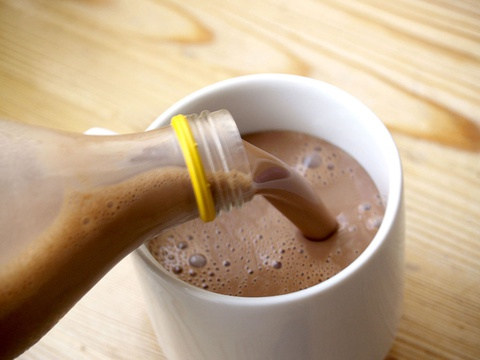# Double Number Line for Modeling# Model With Mathematics

• Watch the first video, which introduces the problem in Protein Shake Mistake.
• Can you explain what the problem is asking?
• Then watch the second video, in which students use ratios to model the mathematics in the problem.
• How did Carlos, Denzel, and Jan model the protein shake problem?
• How did they adjust the model to determine the amount they needed?

VIDEO: Protein Shake Mistake

VIDEO: Model with Mathematics

1 of 9